# TURBO CODED SIGNALING over a MEMORY CHANNEL

NOTE: This section is Under-Edit if necessary: Construction began on June 18, 2017 and was finished on June 18, 2017.

Turbo Coded Signaling over a Memory Channel: M-ary Modulation Schemes, Rayleigh Fading, and the Iterative Turbo Decoder

```by Darrell A. Nolta
June 18, 2017
```

The AdvDCSMT1DCSS Professional (T1 Version 2) system tool provides the capability to model and simulate Multiple Iteration Soft Input/Soft-Decision Output (SISO) Turbo Code (TC) Channel Decoding using a Turbo Decoding Algorithm where two SISO 'symbol-by-symbol' Maximum a Posteriori Probability (MAP) Algorithm component decoders (DEC1 & DEC2) are serial concatenated with a feedback loop. This decoding algorithm can be used in the cases for Turbo Coded Signaling over Memory Channels (MemC) with Additive White Gaussian Noise (AWGN).

This capability of T1 Professional supports decoding of Turbo Coded M-ary Signaling over a Gilbert-Elliot (GE) Burst Channel, Fading Channel (Rayleigh, Rician, and Frequency-Selective), or Intersymbol Interference (ISI) Channel.

T1 V2 provides the User the ability to add a Symbol Interleaver-DeInterleaver for the GE Burst Channel or Fading Channel Signaling simulations. In a Fading Channel simulation, a Diversifier-Combiner can be used instead of the Symbol Intlerleaver-DeInterleaver. In an ISI Channel simulation, a Linear Equalizer can be added.

Also, this capability of T1 Professional supports decoding of TC Signaling over a Classic Bursty Channel (CBC) with background error. In a CBC simulation, a Block Interleaver-DeInterleaver can be used.

In addition, T1 Professional provides the capability to model and simulate a Non-Iterative (Single Iteration) SISO TC Channel Decoding where DEC1 and DEC2 are serial concatenated without the feedback loop.

And this new feature provides the capability to puncture the Turbo Code so that the Turbo Code Rate is increased. One can achieve a Punctured Rate Rp of 1/2 for a parent TC Rate of 1/3; Rp of 1/2, 1/3, or 1/4 for a parent TC Rate of 1/5; and Rp of 1/2, 1/3, 1/4, 1/5, or 1/6 for a parent TC Rate of 1/7.

This paper will focus on the application of T1 V2 in the study of the use of Turbo Coding and Decoding to reduce the Information Bit Errors caused by Rayleigh Fading. Rayleigh Fading is a very important channel impairment that is very destructive in a wireless/mobile communication system that attempts to signal over a dispersive (multipath) environment.

Rayleigh fading channel is a flat (non-selective) fading channel whose parameters vary in time that applies a multiplicative complex Gaussian noise on the transmitted signal. The Rayleigh Fading Channel is a Memory Channel where the physical state of this channel can be characterized by the fading level of a slowly fading transmission path (Robert G. Gallager , page 97). It is important to realize that this channel does not act on each of its inputs in a statistically independent manner. This channel is destructive of the phase information contained within the symbol-to-symbol detection of the channel output signal (i.e., phase variation of the transmitted/received signal). Coherent detection/demodulation cannot be use as long as this phase information cannot be recovered from the received signal.

Given the nature of the Rayleigh Fading Channel, the Frequency Shift Keying (FSK), an orthogonal modulation, is the only modulation to be use for this channel as long as noncoherent demodulation is the only possibility and if the required signal bandwidth is not a limiting factor.

Thus, T1 V2 will offer only FSK modulation when the User selects the Fading Channel for a simulation. And, for the Fading Channel selection, the Turbo Decoder uses Energy Detector Demodulation with Fading Statistics.

Consider the Turbo Decoding Algorithm Bit Error Rate (BER) or Bit Error Probability Pb performance simulation results that were produced by T1 V2 that are displayed below in Figure 1, 2, 3, 4, 5, 6, 7, and 8 plots. Also, in addition to the Pb results, Figure 7 and 8 displays the corresponding Average Number of Iterations per Block (Avg I).

For comparison purposes, simulated BER results for UnCoded and Convolutional Coded (CC) (Rate = 1/2 or 1/3, 8-State) BFSK, 4-FSK, and 8-FSK Signaling are included appropriately in these figures. Maximum Likelihood (ML) Energy Detector Demodulation without Fading Statistics is used for UnCoded BFSK, 4-FSK, or 8-FSK Signaling. And ML Energy Detector Demodulation without Fading Statistics and Viterbi Algorithm Decoding is used for CC BFSK, 4-FSK, or 8-FSK Signaling.

Each figure's BER plot displays one or more curves where a curve is constructed from the set of simulated Pb values that correspond to a set of Eb/N0 [Signal-to-Noise Ratio (SNR)] values in dB. Each Turbo Coding (TC) and Punctured Turbo Coding (PTC) curve displays the performance behavior of a TC and PTC and Iterative Decoding system example that was used for a T1 V2 simulation.

Figure 1 displays the BER versus Eb/N0 for One Iteration and Cross-Entropy (CE) Stopping Rule (SR) Iterative Decoding of Rate = 1/3 Turbo Coded BFSK Signaling for 1,001,472 Equal probable i.i.d. Information (Info) Bits.

Figure 2 displays the BER versus Eb/N0 for One Iteration and CE Stopping Rule Iterative Decoding of Rate = 1/2 Punctured Turbo Coded BFSK Signaling for 1,001,472 Equal probable i.i.d. Info Bits.

Figure 3 displays the BER versus Eb/N0 for One Iteration and CE Stopping Rule Iterative Decoding of Rate = 1/3 Turbo Coded 8-FSK Signaling for 1,001,472 Equal probable i.i.d. Info Bits.

Figure 4 displays the BER versus Eb/N0 for One Iteration and CE Stopping Rule Iterative Decoding of Rate = 1/2 Punctured Turbo Coded 4-FSK Signaling for 1,001,472 Equal probable i.i.d. Info Bits.

Figure 5 displays BER versus Eb/N0 for CE Stopping Rule Iterative Decoding of Rate = 1/3 Turbo Coded and Rate = 1/2 Punctured Turbo Coded BFSK Signaling for 1,001,472 and 1,001,472 Equal probable i.i.d. Info Bits.

Figure 6 displays BER versus EbN0 for CE Stopping Rule Iterative Decoding of Rate = 1/3 Turbo Coded 8-FSK Signaling and Rate = 1/2 Punctured Turbo Coded 4-FSK Signaling for 1,001,472 and 1,001,472 Equal probable i.i.d. Info Bits.

Figure 7 displays BER and Avg I versus Eb/N0 for CE Stopping Rule Iterative Decoding of Rate = 1/3 Turbo Coded 8-FSK Signaling for 1,001,472 Equal probable i.i.d. Info Bits.

Figure 8 displays BER and Avg I versus Eb/N0 for CE Stopping Rule Iterative Decoding of Rate = 1/2 Punctured Turbo Coded 4-FSK Signaling for 1,001,472 Equal probable i.i.d. Info Bits.

There are a number of important observations that can be drawn from the below displayed simulated Iterative Turbo Code (ITC) and Punctured Turbo Code (IPTC) Decoding BER results. It appears that T1 is correctly modeling and simulating Turbo Coded and Punctured Turbo Coded Signaling over a Rayleigh Fading Channel (Memory Channel) with AWGN with Iterative Decoding for the selected Turbo and Punctured Turbo Codes.

We can clearly observe in Figure 1 the dramatic reduction in BER (~2x10-1 to ~7x10-5) in the low SNR region (known as the 'waterfall') for the TC BFSK Signaling with ITC Decoding [CE Stopping Rule (SR)] as compared to the UnCoded BFSK or CC BFSK Signaling with VA Decoding or TC One Iteration ITC Decoding. After reaching the waterfall BER minimum, the BER curve begins its oscillation as SNR increases (15 dB to 25 dB). Eventually, the BER reaches ~4x10-5 at SNR equal to 25 dB. In regards to the One Iteration ITC Decoding BER curve, the BER decreases gracefully with increasing SNR and then levels off. But what is most interesting is that in the 'high SNR' region, the BER values corresponding to the CE SR ITC Decoding is greater than the BER values corresponding to the One Iteration ITC Decoding. And at 'high SNR', CC and Viterbi Algorithm Decoding BER values are less than the ITC Decoding of TC BFSK Signaling.

In Figure 2 (PTC BFSK Signaling with IPTC Decoding), we can see the same BER behavior as seen in Figure 1 except the CE SR ITC Decoding curve 's 'waterfall' BER minimum is larger (~1x10-4 at 16 dB vs. ~7x10-5 at 15 dB).

In Figure 3 (TC 8-FSK Signaling with ITC Decoding), we can view the same BER behavior as seen in Figure 1 except the CE SR ITC Decoding curve's 'waterfall' BER minimum occurs at 12 dB instead of 15 dB. From SNR of 12 to 15 dB, the BER curve continues to decline gracefully. From SNR of 15 dB to 25 dB, the BER curve flatten at ~1x10-5.

In Figure 4 (PTC 4-FSK Signaling with IPTC Decoding), we can view the same BER behavior as seen in Figure 2 except one can see two 'waterfall' BER segments of the CE SR IPTC Decoding curve ('waterfall' BER minimums at SNR equal to 14 dB & 19 dB).

In Figure 5 (CE SR ITC Decoding for TC BFSK Signaling vs. CE SR IPTC Decoding for PTC BFSK Signaling), we can observe that the 'waterfall' BER segment for TC BFSK occurs at a smaller SNR than for PTC BFSK. But in the SNR region of 22 dB to 25dB, the PTC BFSK BER curve is identical statistically to the TC BFSK BER curve.

In Figure 6 (CE SR ITC Decoding for TC 8-FSK Signaling vs. CE SR IPTC Decoding for PTC 4-FSK Signaling), we can see that the 'waterfall' BER segment for TC 8-FSK occurs at a smaller SNR than for PTC 4-FSK. But in the SNR region of 19 dB to 25dB, the PTC BFSK BER curve is identical statistically to the TC BFSK BER curve.

In Figure 7 (CE SR ITC Decoding for TC 8-FSK Signaling), we can view that the 'waterfall' BER segment occurs when the Average Number of Iterations (Avg I) drops from 5 to ~4.8 ('waterfall' BER minimum occurs at SNR equal to 12 dB). The Avg I plummets from ~4.8 to ~2.1 at SNR equal to 15 dB.

In Figure 8 (CE SR IPTC Decoding for PTC 4-FSK Signaling), we can view that the 'waterfall' BER segment occurs when the Average Number of Iterations (Avg I) drops from 5 to ~4.6 ('waterfall' BER minimum occurs at SNR equal to 14 dB). The Avg I plummets from ~4.6 to ~2.1 at SNR equal to 19 dB.

Based on these BER results displayed in Figures 1 through 8, the BER behavior of Turbo Decoding of Turbo Coded/Punctured Turbo Coded Signaling over a Rayleigh Fading Channel (a Memory Channel) with AWGN appears to exhibit the known BER behavior of Turbo Decoding of Turbo Coded/Punctured Turbo Coded Signaling over a coherent Memoryless Channel (MLC) with AWGN [i.e., as the number of iterations is increased (from one iteration to multiple iterations), the SNR corresponding to the 'waterfall' BER minimum is reduced (i.e., moving toward the Shannon Limit)].

But how is this possible?

As a reminder, let us review the basic underlying algorithm applied by each component decoder (DEC1 & DEC2) of a Turbo Decoder. This algorithm is based on the BCJR algorithm that is described by L.R. Bahl, J. Cocke, F. Jelinek, & J. Raviv. in their 1974 paper . Each component decoder implements a SISO 'symbol-by-symbol' Maximum a Posteriori Probability (MAP) Algorithm. Each component decoder's MAP Algorithm calculates the a posteriori probability L-value (natural log likelihood ratio) for each Information Bit. The Turbo Decoder's component MAP decoder is using a metric that is based on sums and not on products of likelihood ratios of a MLC representation,

The purpose of the BCJR algorithm is to estimate optimally the a posteriori probability (APP) of each Information Bit that is transmitted. It is important to realize that the BCJR algorithm is based on the definition that the probability of a particular channel output sequence is a product of individual transition probabilities, i.e., these individual transition probabilities are statistically independent of each other. A MLC satisfies this definition. An AWGN channel is a MLC. The memory channel does not satisfy this definition: the transition probabilities are not statistically independent and, thus, the product of these individual probabilities does not represent the channel output sequence probability.

The Rayleigh Fading Channel without AWGN is a Memory Channel. When AWGN is added to the Rayleigh Fading Channel, the channel output would appear to become statistically independent. This fact may explain why Turbo Decoding achieves such good BER results for the Rayleigh Fading Channel with AWGN. We must observe the fact that it is impossible practically to transmit information over a Rayleigh Fading Channel without AWGN. But these BER results are not optimal because the Turbo Decoder (serial concatenated component decoders with a feedback loop) is not an optimal decoder and the Non-Coherent Demodulator does not take into consideration the Markov State structure of the Rayleigh Fading Channel.

T1 Professional (T1 V2) now offers the Turbo Channel Coding and Turbo Channel Decoding (Iterative & Non-Iterative; based on the 'Symbol-by-Symbol' MAP algorithm) for Memory Channel features to the User. One can choose to puncture the Turbo Code. The User can choose the simulated Signaling Channel as the Classic Bursty Channel (CBC) with background error or Gilbert-Elliot Burst Channel (GEBC); Rayleigh, Rician, or Frequency-Selective Fading Channel; or PAM or QAM InterSymbol Interference Channel with Linear Equalizer. A Block Interleaver-DeInterleaver (Intl-DeIntl) can be used with CBC or a Symbol Intl-DeIntl can be used with GEBC or Fading Channel. A Diversifier -Combiner can be used for a simulation of Signaling over a Fading Channel.

In conclusion, the User via T1 V2 can get experience with these algorithms as applied to Iterative Decoding in simulated digital communication systems for Spacecraft and Mobile Communications Turbo Coding applications.

References:

 Robert G. Gallager, Information Theory and Reliable Communication, John Wiley & Sons, New York, 1968.

 L.R. Bahl, J Cocke, F. Jelinek, and J. Raviv, "Optimal Decoding of Linear Codes for Minimizing Symbol Error Rate," IEEE Transactions on Information Theory, Vol. 20, pp. 284-287, March 1974.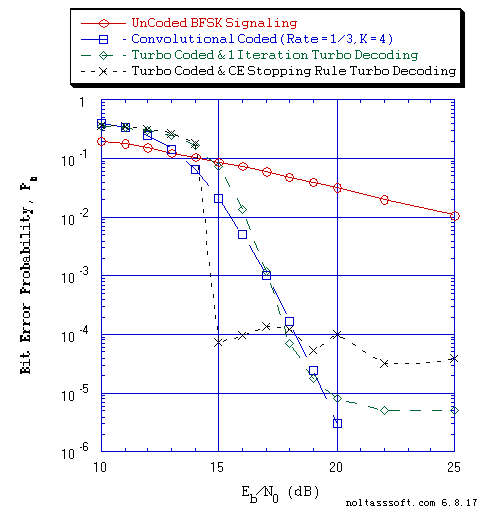```Figure 1. Bit Error Probability for UnCoded, Convolutional, and Turbo Coded BFSK
White Gaussian Noise (AWGN):
```
```          Equal probable Independent and Identical Distributed  (IID) Source for 10
Million, 1 Million, and 1,001,472 Information Bits for UnCoded,
Convolutional Coded, and Turbo Coded BFSK Signaling respectively over a
Vector Channel;
```
```          Rate = 1/3, Constraint Length K = 4, (7,5,6,7), a Best (Optimal)
Non-Recursive Convolutional Code (J.P. Odenwalder) and Viterbi Algorithm
using a Path Memory Length of 20 bits and an Unquantized Branch Metric;
```
```          Rate (r) = 1/3, K = 4 Turbo Code based on a r = 1/2, K = 3 (G0 = 13
octal, G1 = 17 octal) Recursive Systematic Convolutional component code
(Optimum-Weight Spectrum) and a 6144-Bit QPP Turbo Encoder Interleaver;
```
```          Rayleigh Fading Channel: Received Information Baseband SNR Loss due to
```
```          Maximum Likelihood (ML) Energy Detector Demodulation; and
```
```          Max-Log-MAP Iterative Turbo Decoder using Systematic Channel Output as
input to both component decoders and Decode Information Bit Decision is
based on the Sum of both decoders' log-likelihood ratios (L-values) and for
One Iteration and Cross-Entropy (CE) Stopping Rule (Five Iterations Max).
```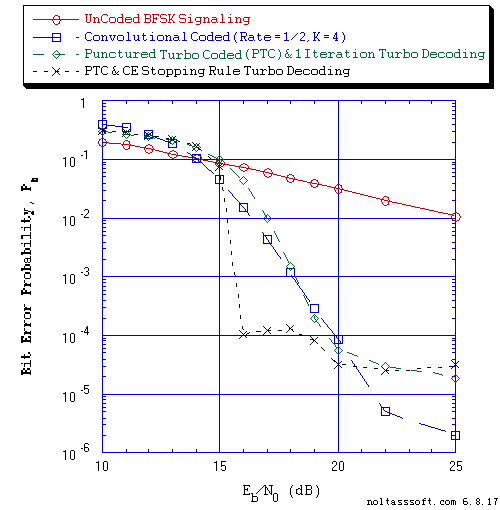```Figure 2. Bit Error Probability for UnCoded, Convolutional, and Punctured Turbo
Coded (PTC) BFSK Signaling over a Rayleigh Fading Memory Channel
with Additive White Gaussian Noise (AWGN):
```
```          Equal probable Independent and Identical Distributed (IID) Source for
10 Million, 1 Million, and 1,001,472 Information Bits for UnCoded,
Convolutional Coded and PTC BFSK Signaling respectively over a
Vector Channel;
```
```          Rate = 1/2, Constraint Length K = 4, (3,2,3,3), a Best (Optimal)
Non-Recursive Convolutional Code (J.P. Odenwalder) and Viterbi Algorithm
using a Path Memory Length of 20 bits and an Unquantized Branch Metric;
```
```          Rate (r) = 1/3, K = 4 Parent Turbo Code based on a r = 1/2, K = 4 (G0 = 13
octal, G1 = 17 octal) Recursive Systematic Convolutional component code
(Optimum-Weight Spectrum) and a 6144-Bit QPP Turbo Encoder Interleaver;
```
```          Punctured Turbo Code Rate = 1/2 for Puncturing Period of 2 and Puncturing
Matrix = [[1,1],[1,0],[0,1]];
```
```          Rayleigh Fading Channel: Received Information Baseband SNR Loss due to
```
```          ML Energy Detector Demodulation; and
```
```          Max-Log-MAP Iterative Turbo Decoder using Systematic Channel Output as
input to both component decoders and Decoder Information Bit Decision is
based on the Sum of both decoders' log-likelihood ratios (L-values) and for
One Iterations and Cross-Entropy (CE) Stopping Rule (Five Iterations Max).
```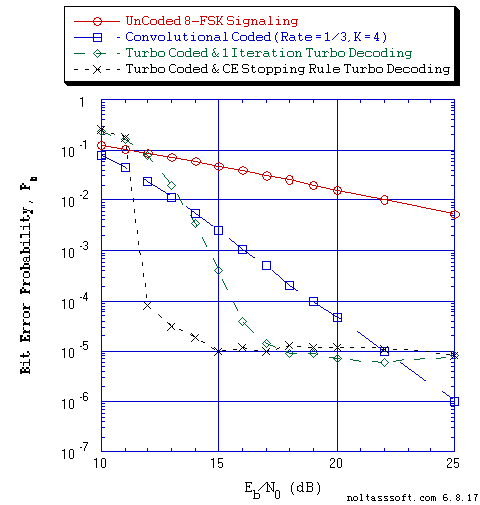```Figure 3. Bit Error Probability for UnCoded, Convolutional, and Turbo Coded
White Gaussian Noise (AWGN):
```
```          Equal probable Independent and Identical Distributed  (IID) Source for
10,000,002, 1 Million, and 1,001,472 Information Bits for UnCoded,
Convolutional Coded, and Turbo Coded 8-FSK Signaling respectively over a
Vector Channel;
```
```          Rate = 1/3, Constraint Length K = 4, (7,5,6,7), a Best (Optimal)
Non-Recursive Convolutional Code (J.P. Odenwalder) and Viterbi Algorithm
using a Path Memory Length of 20 bits and an Unquantized Branch Metric;
```
```          Rate (r) = 1/3, K = 4 Turbo Code based on a r = 1/2, K = 3 (G0 = 13
octal, G1 = 17 octal) Recursive Systematic Convolutional component code
(Optimum-Weight Spectrum) and a 6144-Bit QPP Turbo Encoder Interleaver;
```
```          Rayleigh Fading Channel: Received Information Baseband SNR Loss due
```
```          8-FSK Demodulation Constellation DeMapper Algorithm: Max-Log Bit Metrics
& ML Energy Detector; and
```
```          Max-Log-MAP Iterative Turbo Decoder using Systematic Channel Output as
input to both component decoders and Decode Information Bit Decision is
based on the Sum of both decoders' log-likelihood ratios (L-values) and for
One Iteration and Cross-Entropy (CE) Stopping Rule (Five Iterations Max).
``````Figure 4. Bit Error Probability for UnCoded, Convolutional, and Punctured
Turbo Coded (PTC) 4-FSK Signaling over a Rayleigh Fading Memory Channel
with Additive White Gaussian Noise (AWGN):
```
```          Equal probable Independent and Identical Distributed (IID) Source for 10
Million, 1 Million, and 1,001,472 Information Bits for UnCoded,
Convolutional Coded and PTC 4-FSK Signaling respectively over a
Vector Channel;
```
```          Rate = 1/2, Constraint Length K = 4, (3,2,3,3), a Best (Optimal)
Non-Recursive Convolutional Code (J.P. Odenwalder) and Viterbi Algorithm
using a Path Memory Length of 20 bits and an Unquantized Branch Metric;
```
```          Rate (r) = 1/3, K = 4 Parent Turbo Code based on a r = 1/2, K = 4 (G0 = 13
octal, G1 = 17 octal) Recursive Systematic Convolutional component code
(Optimum-Weight Spectrum) and a 6144-Bit QPP Turbo Encoder Interleaver;
```
```          Punctured Turbo Code Rate = 1/2 for Puncturing Period of 2 and
Puncturing Matrix = [[1,1],[1,0],[0,1]];
```
```          Rayleigh Fading Channel: Received Information Baseband SNR Loss due
```
```          4-FSK Demodulation Constellation DeMapper Algorithm: Max-Log Bit Metrics
& ML Energy Detector; and
```
```          Max-Log-MAP Iterative Turbo Decoder using Systematic Channel Output as
input to both component decoders and Decoder Information Bit Decision is
based on the Sum of both decoders' log-likelihood ratios (L-values) and for
One Iterations and Cross-Entropy (CE) Stopping Rule (Five Iterations Max).
```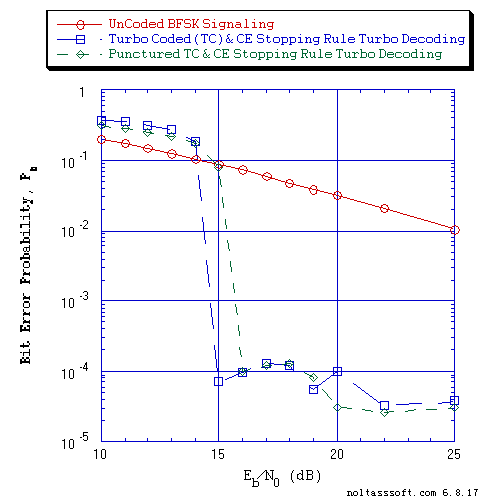```Figure 5. Bit Error Probability for UnCoded, Turbo, and Punctured
Turbo Coded (PTC) BFSK Signaling over a Rayleigh Fading Memory Channel
with Additive White Gaussian Noise (AWGN):
```
```          Equal probable Independent and Identical Distributed  (IID) Source for 10
Million, 1,001,472, and 1,001,472 Information Bits for UnCoded, Turbo
Coded, and PTC BFSK Signaling respectively over a Vector Channel;
```
```          Rate (r) = 1/3, K = 4 Turbo Code based on a r = 1/2, K = 3 (G0 = 13
octal, G1 = 17 octal) Recursive Systematic Convolutional component code
(Optimum-Weight Spectrum) and a 6144-Bit QPP Turbo Encoder Interleaver;
```
```          Punctured Turbo Code Rate = 1/2 for Puncturing Period of 2 and
Puncturing Matrix = [[1,1],[1,0],[0,1]];
```
```          Rayleigh Fading Channel: Received Information Baseband SNR Loss due
```
```          ML Energy Detector Demodulation; and
```
```          Max-Log-MAP Iterative Turbo Decoder using Systematic Channel Output as
input to both component decoders and Decode Information Bit Decision is
based on the Sum of both decoders' log-likelihood ratios (L-values) and
for Cross-Entropy (CE) Stopping Rule (Five Iterations Max).
```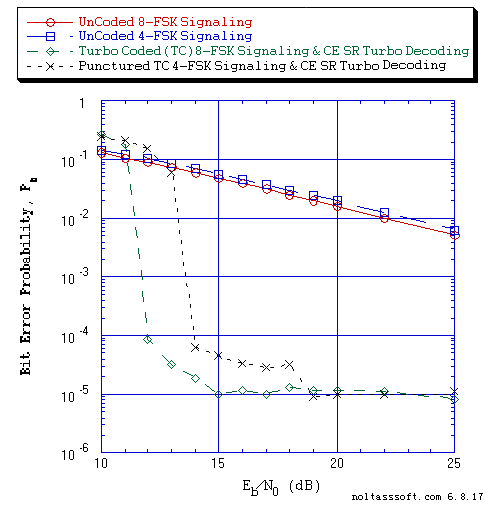```Figure 6. Bit Error Probability for UnCoded 8-FSK & 4-FSK, Turbo Coded 8-FSK, and
Punctured Turbo Coded (PTC) 4-FSK Signaling over a Rayleigh Fading Memory
Channel with Additive White Gaussian Noise (AWGN):
```
```          Equal probable Independent and Identical Distributed  (IID) Source for
10,000,002 & 10 Million; 1,001,472, and 1,001,472 Information Bits for
UnCoded 8-FSK & 4-FSK; Turbo Coded 8-FSK, and PTC 4-FSK Signaling
respectively over a Vector Channel;
```
```          Rate (r) = 1/3, K = 4 Turbo Code based on a r = 1/2, K = 3 (G0 = 13
octal, G1 = 17 octal) Recursive Systematic Convolutional component code
(Optimum-Weight Spectrum) and a 6144-Bit QPP Turbo Encoder Interleaver;
```
```          Punctured Turbo Code Rate = 1/2 for Puncturing Period of 2 and
Puncturing Matrix = [[1,1],[1,0],[0,1]];
```
```          Rayleigh Fading Channel: Received Information Baseband SNR Loss due
```
```          8-FSK Demodulation Constellation DeMapper Algorithm: Max-Log Bit Metrics
& ML Energy Detector;
```
```          4-FSK Demodulation Constellation DeMapper Algorithm: Max-Log Bit Metrics
& ML Energy Detector; and
```
```          Max-Log-MAP Iterative Turbo Decoder using Systematic Channel Output as
input to both component decoders and Decode Information Bit Decision is
based on the Sum of both decoders' log-likelihood ratios (L-values) and for
Cross-Entropy (CE) Stopping Rule (Five Iterations Max).
```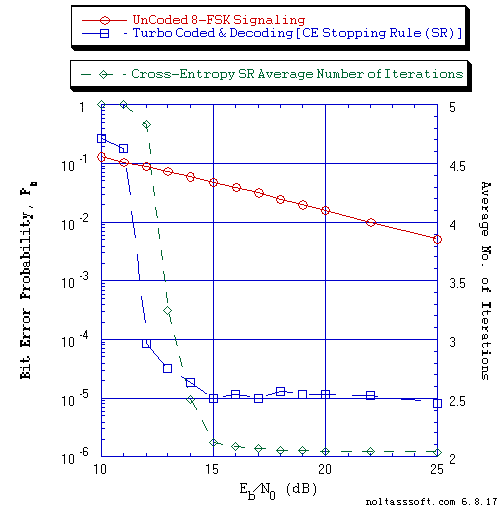```Figure 7. Bit Error Probability for UnCoded and Turbo Coded 8-FSK Signaling over
```
```          Equal probable Independent and Identical Distributed  (IID) Source for
10,000,002, and 1,001,472 Information Bits for UnCoded, and Turbo
Coded 8-FSK Signaling respectively over a Vector Channel;
```
```          Rate (r) = 1/3, K = 4 Turbo Code based on a r = 1/2, K = 3 (G0 = 13
octal, G1 = 17 octal) Recursive Systematic Convolutional component code
(Optimum-Weight Spectrum) and a 6144-Bit QPP Turbo Encoder Interleaver;
```
```          Rayleigh Fading Channel: Received Information Baseband SNR Loss due
```
```          8-FSK Demodulation Constellation DeMapper Algorithm: Max-Log Bit
Metrics & ML Energy Detector; and
```
```          Max-Log-MAP Iterative Turbo Decoder using Systematic Channel Output as
input to both component decoders and Decode Information Bit Decision is
based on the Sum of both decoders' log-likelihood ratios (L-values) and
for Cross-Entropy (CE) Stopping Rule (Five Iterations Max).
```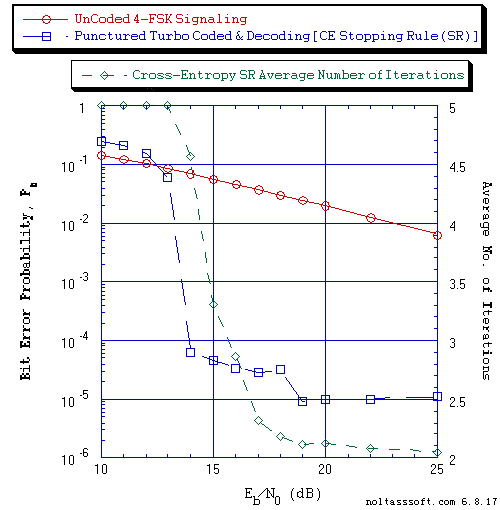```Figure 8. Bit Error Probability for UnCoded and Punctured Turbo Coded
White Gaussian Noise (AWGN):
```
```          Equal probable Independent and Identical Distributed (IID) Source for 10
Million and 1,001,472 Information Bits for UnCoded and PTC 4-FSK Signaling
respectively over a Vector Channel;
```
```          Rate (r) = 1/3, K = 4 Parent Turbo Code based on a r = 1/2, K = 4 (G0 = 13
octal, G1 = 17 octal) Recursive Systematic Convolutional component code
(Optimum-Weight Spectrum) and a 6144-Bit QPP Turbo Encoder Interleaver;
```
```          Punctured Turbo Code Rate = 1/2 for Puncturing Period of 2 and
Puncturing Matrix = [[1,1],[1,0],[0,1]];
```
```          Rayleigh Fading Channel: Received Information Baseband SNR Loss due
```          4-FSK Demodulation Constellation DeMapper Algorithm: Max-Log Bit Metrics
```          Max-Log-MAP Iterative Turbo Decoder using Systematic Channel Output as